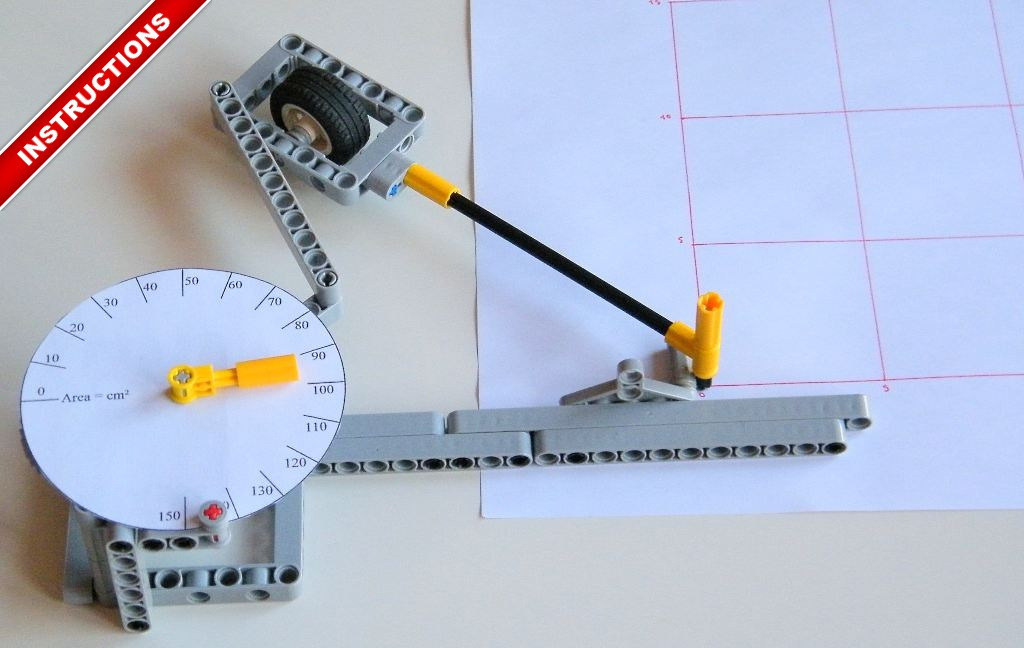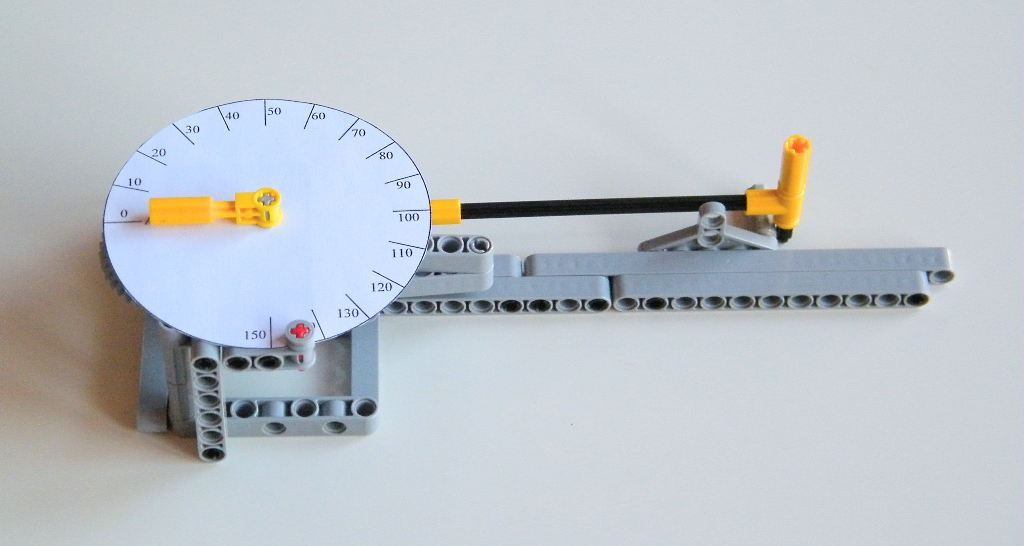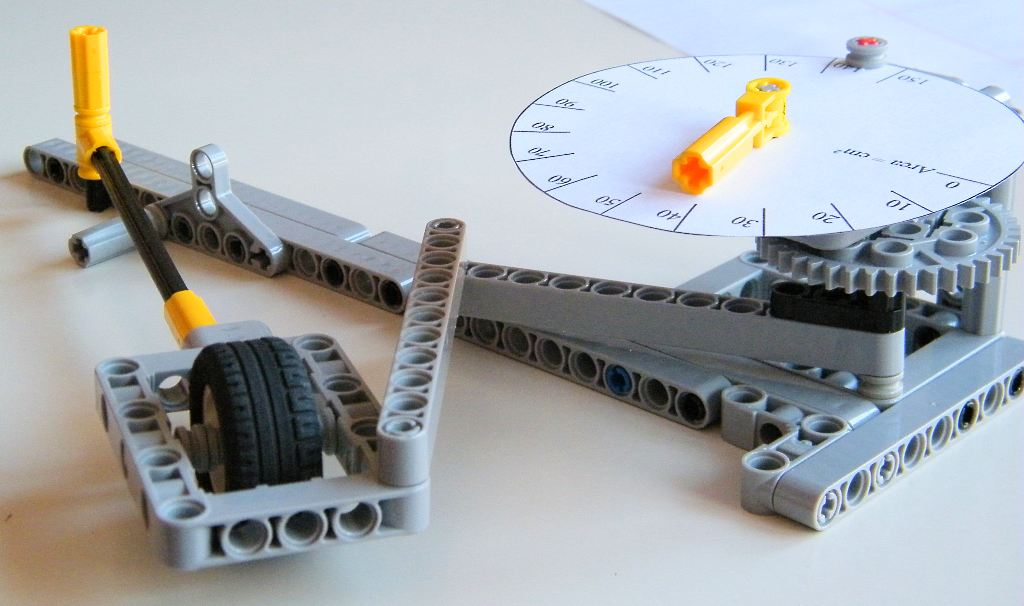My first planimeter, very simple and very demonstrative to understand how a mechanical device can calculate area.A planimeter is a device which is used to calculate surface and area from a drawing of the shape. By traveling the boundary of the shape, the measuring instrument integrates the area of the shape which is equal of the surface in the shape. This type of planimeter was used to calculate area on a mathematical curve, boundary of a country or measure and increase efficiency of machines for instance. There are two type of planimeters, those which work with precisely integrator with a mechanical device, and those which estimate the area with a approximation. The Pritz planimeter, also caller Hatchet, is a second type planimeter.My planimeter is a modified version of a pritz planimeter which is very simple. It is composed of several elements :

• The main rod, which is connected to the wheel and the cursor
• The wheel which can roll but not slide
• A connecting rods system which links the angle of the main rod on a gearing (addition)
• The gearing up (40/8) which increases the angular motion (addition)
• A dial which shows the final measure (addition)

Now how does it works ? With the cursor, you travel the boundaries of the shape, and after comes back to the starting point, the planimeter shows the measure. Mathematically, the planimeter use a tractrix which approximate the derivative necessary to calculate the area and the Green’s theorem which links the line integral around a simple closed curve and a double integral over the plane region. Consequently the area is roughly equal to the difference between the starting and final angular position of the main rod.To understand more precisely  you can read the description of the planimeter on this website and the maths demonstration and the Green’s theorem.

This device is not very accurate, because the derivative is approximate by a tractrix and because the connecting rod system is not very linear. With low or high value, the non-linearity of the system causes interference with the measure. In any case, it is a simple and very demonstrative of the genius of ancient engineers to measure things with mechanical device.

I have made the building instructions, I encourage you to build it to understand this clever device.Downloading the high definition PDF building instructions. Pritz Planimeter by Nico71 – 3mo, PDF